• 图像匹配滤波器Matlab程序 附注释 图像匹配滤波
• 需要注意的是，本次所要讲的内容是内插滤波器(Interpolated FIR,IFIR)的FPGA实现 ,而不是我们熟知的插值滤波器(Interpolaton FIR)。首先来介绍一下内插滤波器的结构原理和作用。内插滤波器(IFIR)和传统的 FIR 滤波器...


需要注意的是，本次所要讲的内容是内插滤波器(Interpolated FIR,IFIR)的FPGA实现 ,而不是我们熟知的插值滤波器(Interpolaton FIR)。首先来介绍一下内插滤波器的结构原理和作用。内插滤波器(IFIR)和传统的 FIR 滤波器或者插值滤波器有类似的结构，唯一的区别就是将单位延迟替换为了 k -1个延迟单元，其中 k 称为 0填充因子。 这种结构的滤波器在功能上相当于在原型滤波器的系数集的系数之间插入了k-1个零。内插滤波器的最终的数据输出采样率与输入数据采样率一致，而不改变数据采样率。所以内插滤波器(Interpolated FIR,IFIR)对于实现窄带滤波器和宽带滤波器的高效实现是非常有用的。用内插滤波器实现普通窄带FIR滤波器或者宽带FIR滤波器的时候能够节省非常可观的DSP资源。下面是Xilinx 用户手册关于该滤波器的简介，如图1所示。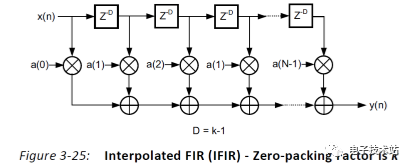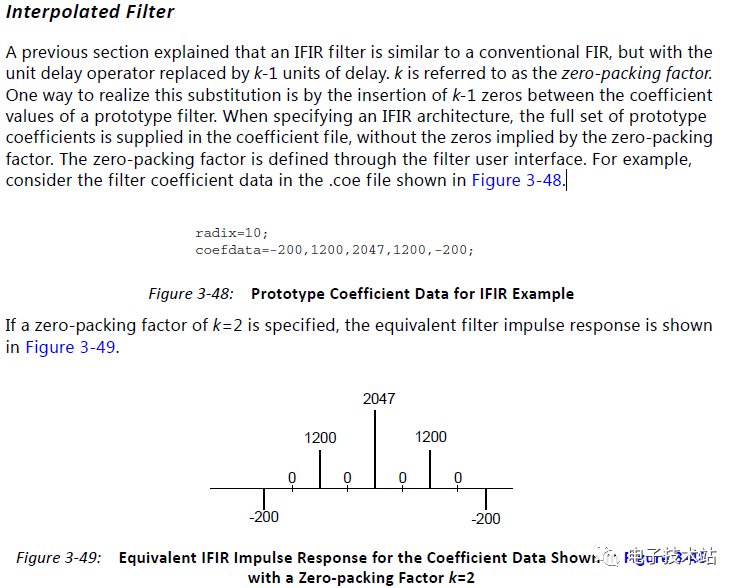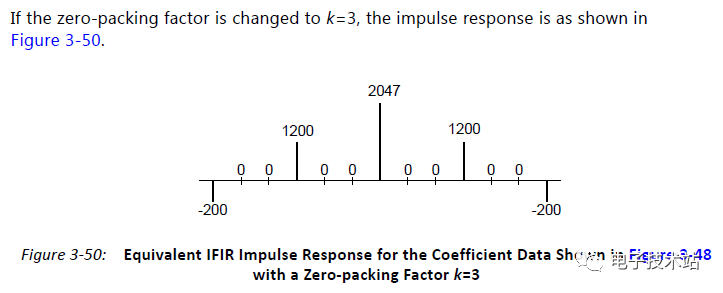图 1该内插滤波器在实现时，Xilinx FPGA开发环境中已经集成了该内插滤波器IP核类型可供我们选择。在指定IFIR体系结构时，在coefficient文件中提供了完整的原型系数集，而不包含零填充因子所暗示的零。零填充因子是通过过滤器用户界面定义的，如图2所示。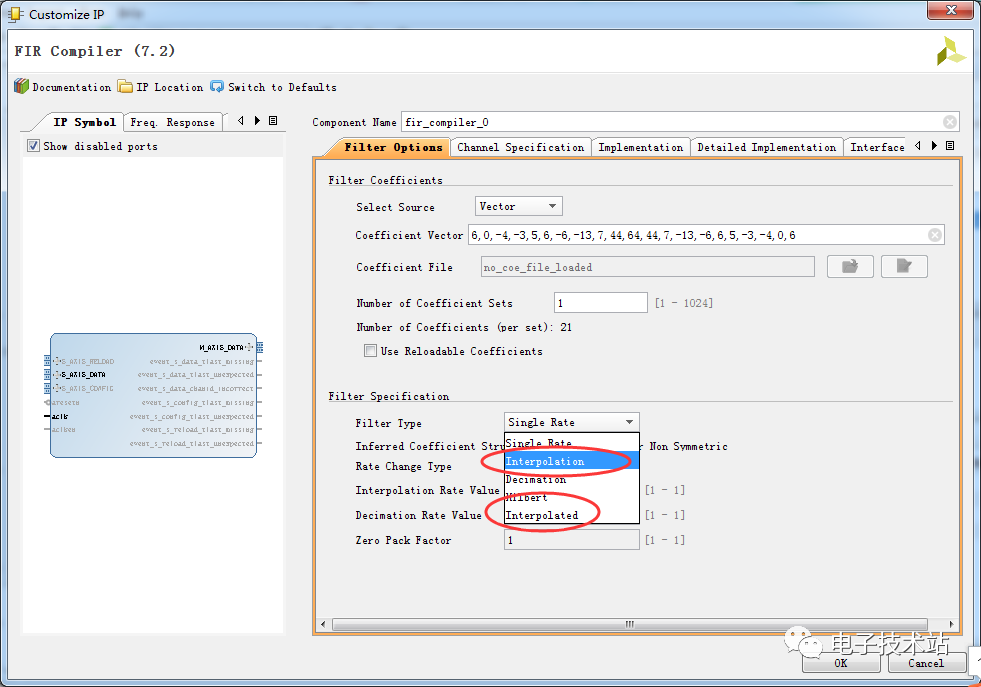图 2        在Xilinx平台实现该内插滤波器时可以通过IP来实现，但是在Intel的Quartus开发环境中没有集成该IP，要想实现该结构的滤波器需要手动的方式编写的方式实现该内插滤波器。因此本文通过Verilog的代码来进行设计实现。设计的内插滤波器工作时钟为300MHz，数据采样率为150MHz，原型滤波器的抽头数为83阶。内插4倍相当于在每个抽头之间插入3个0，相当于328阶的效果。与其他类型的插值滤波器一样，插值会产生镜像频谱。由于工作时钟是数据采样率的两倍，因此可以考虑对乘法器进行复用，可以节省一半的DSP资源。最终只使用21个DSP即可实现。如图3所示。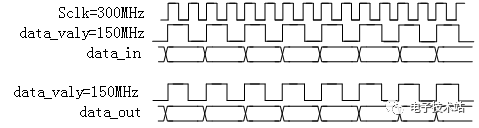图3  这里我设计了一种高通滤波器方案，先通过Matlab设计需要的高通滤波器，并导出抽头系数进行量化为16bit。然后如图4所示。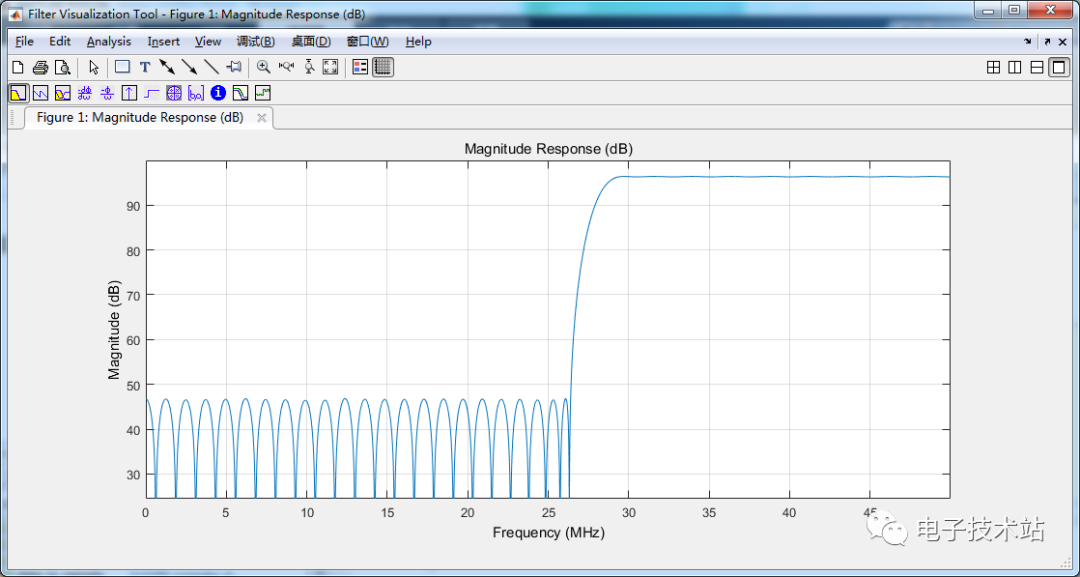图4       这里我们是通过对称加法树结构来进行编写Verilog代码，并对加法树的输出进行分组复用。部分代码如图5所示。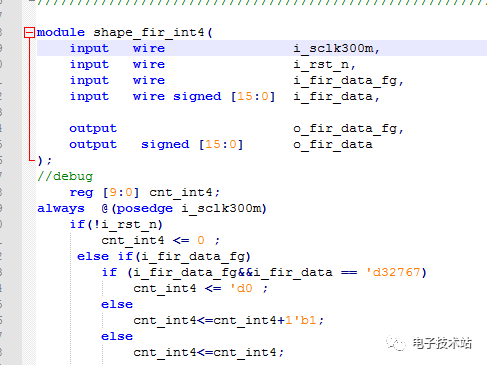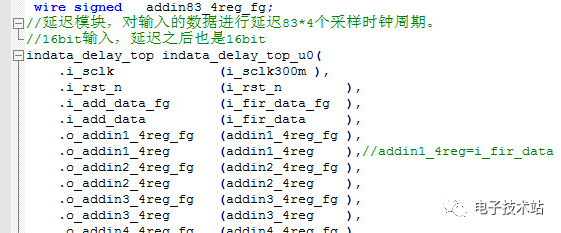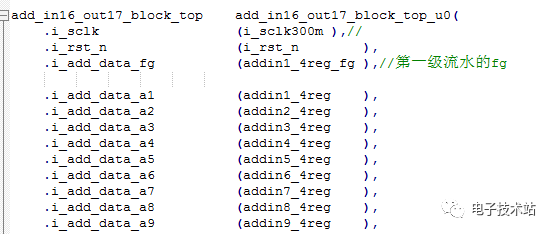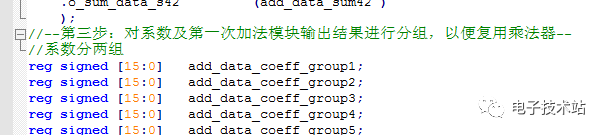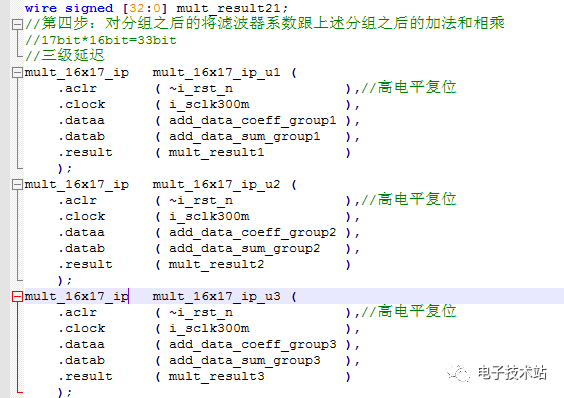图5       最终通过输入一组点频数据源通过Modelsim仿真可以得到输出的结果。如图6所示。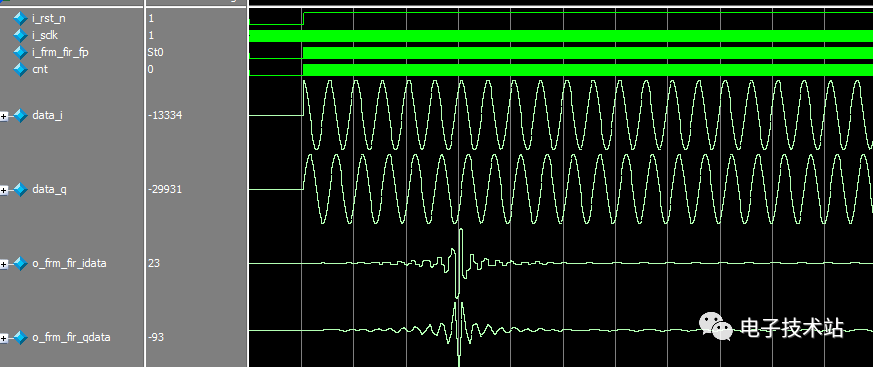对Modelsim仿真输出的数据进行Matlab画图可以得到内插滤波器的频谱响应为，图7所示。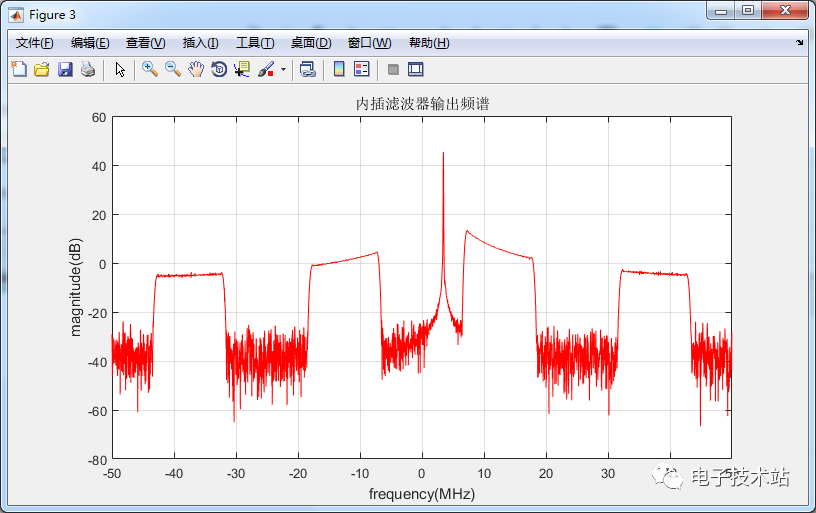图7       此时通过频谱图我们可以得知，数据经过4倍内插滤波器之后，输出的数据产生了镜像频谱，后续可以通过叠加滤波器的方式来进行滤除，并可以根据你所需要的效果定制所需的滤波器结构。    下次我们分享一下，通过这种内插滤波器的方式设计其他宽带滤波器的方案，该种设计方案使得我们能够采用最少的DSP资源获得与高阶滤波器等效的效果。

展开全文• ## 匹配滤波器及matlab仿真

千次阅读 多人点赞 2020-06-03 15:28:15
随机信号处理笔记：匹配滤波器 ——南京理工大学顾红老师的《随机信号处理》浅析 文章目录随机信号处理笔记：匹配滤波器1.线性滤波器输出端信噪比2....匹配滤波器的信号处理SNR增益4.1matlab仿真匹配
随机信号处理笔记：匹配滤波器

——南京理工大学顾红老师的《随机信号处理》浅析

文章目录随机信号处理笔记：匹配滤波器1.线性滤波器输出端信噪比2.匹配滤波器的传输函数和冲激响应2.1复函数的施瓦兹不等式2.2传输函数求解3.匹配滤波器的性质3.1匹配滤波器的最大峰值信噪比3.2匹配滤波器的幅频特性相频特性3.3匹配滤波器的物理可实现性3.4输出信号和噪声3.5匹配滤波器的时延适应性3.6匹配滤波器的频移不适应性3.7输出信号频谱与输入信号频谱关系4.匹配滤波器的信号处理SNR增益4.1matlab仿真匹配滤波增益4.1.1理论值：4.1.2仿真图片：4.1.3匹配滤波增益：4.1.4仿真程序：
引言
无线电设备在传输信号时必定伴有噪声。通常，用信号和噪声的功率之比$S/N$表征噪声对信号传输的影响。匹配滤波器理论至今仍是信号检测理论的重要组成部分。

匹配滤波器(matched filter)：白噪声背景中，按照最大信噪比准则，获得最大输出信噪比的线性滤波器。
1.线性滤波器输出端信噪比噪声$n(t)$是零均值的高斯平稳白噪声。其功率谱密度为常量，即：
$G_n(\omega)=\frac{N_0}{2},\qquad -\infty<\omega<\infty$
噪声的自相关函数：
$R(\tau)=E[n(t)n(t+\tau)]=\frac{N_0}{2}\delta(\tau)$
信号$s(t)$的频谱$S(\omega)$为：
$S(\omega)=\int_{-\infty}^{\infty}s(t)e^{-j\omega t}dt$
经过该线性滤波器后，输出信号$s_o(t)$：
$s_o(t)=\frac{1}{2\pi}\int_{-\infty}^{\infty}H(\omega)S(\omega)e^{j\omega t}d\omega$
输出噪声的功率谱密度：
$G_{n_o}(\omega)=G_n(\omega)|H(\omega)|^2=\frac{N_0}{2}|H(\omega)|^2$
进而，输出噪声的平均功率为：
$E[n_o^2(t)]=\sigma^2=\frac{N_0}{4\pi}\int_{-\infty}^{\infty}|H(\omega)|^2d\omega$
$\blacklozenge$ 最后可得到线性滤波器输出端的瞬时信噪比公式：
$SNR= \frac{输出信号的瞬时功率}{输出噪声的平均功率}=\frac{s_o^2(t)}{E[n_o^2(t)]}=\frac{[\frac{1}{2\pi}\int_{-\infty}^{\infty}H(\omega)S(\omega)e^{j\omega t}d\omega]^2}{\frac{N_0}{4\pi}\int_{-\infty}^{\infty}|H(\omega)|^2d\omega }$
$\bigstar$ 假设，在$t=t_0$时刻，线性滤波器输出端输出最大信噪比。此时有：
$SNR_{max}= \frac{输出信号的瞬时功率}{输出噪声的平均功率}=\frac{s_o^2(t_0)}{E[n_o^2(t)]}=\frac{[\frac{1}{2\pi}\int_{-\infty}^{\infty}H(\omega)S(\omega)e^{j\omega t_0}d\omega]^2}{\frac{N_0}{4\pi}\int_{-\infty}^{\infty}|H(\omega)|^2d\omega }$
2.匹配滤波器的传输函数和冲激响应
由式（8）可知，线性滤波器输出的峰值信噪比$SNR_{max}$随系统传输函数$H(\omega)$变化而变化。为寻求最佳的传输函数$H(\omega)$，需要利用复函数的施瓦茨（Schwartz）不等式求解。
2.1复函数的施瓦兹不等式
假设$P(x)$和$Q(x)$都是实变量$x$的复函数，则有如下不等式成立：
$\int_{-\infty}^{\infty}|P(x)|^2dx\int_{-\infty}^{\infty}|Q(x)|^2dx\geq|\int_{-\infty}^{\infty}P^*(x)Q(x)dx|^2$
当且仅当$Q(x)=cP(x)$，不等式取等号。（$c$为常数）
2.2传输函数求解
令$P^*(\omega)=S(\omega)e^{j\omega t_0},Q(\omega)=H(\omega)$，则有：
$|P(\omega)|^2=|S^*(\omega)|^2=|S(\omega)|^2,|Q(\omega)|^2=|H(\omega)|^2$
将式（8）利用施瓦茨不等式改写为：
$SNR_{max}\leq\frac{\frac{1}{4\pi^2}\int_{-\infty}^{\infty}|P(\omega)|^2d\omega\int_{-\infty}^{\infty}|Q(\omega)|^2d\omega}{\frac{N_0}{4\pi}\int_{-\infty}^{\infty}|H(\omega)|^2d\omega } =\frac{\frac{1}{2\pi}\int_{-\infty}^\infty|S(\omega)|^2d\omega}{\frac{N_0}{2}}$
根据巴塞瓦尔能量定理，有：
$\frac{1}{2\pi}\int_{-\infty}^{\infty}|S(\omega)|^2d\omega=\int_{-\infty}^{\infty}s^2(t)dt=E$
其中，$E$：信号输入能量。最终得到如下关系式：
$SNR_{max}\leq\frac{2E}{N_0}$
故，线性滤波器的输出最大信噪比为：$2E/N_0$。（$N_0$是白噪声的单边功率谱密度）
然后根据不等式取等号的条件$Q(\omega)=cP(\omega)$，得到线性系统的传输函数：
$H(\omega) =cS^*(\omega)e^{-j\omega t_0}$

注：转化过程应用了复函数运算重要的性质：复函数乘积的共轭等于各自共轭的乘积。即:$(AB)^*=A^*B^*$(A、B均为复数)

进而利用傅里叶反变换得到线性系统的冲激函数：
$h(t)=\frac{1}{2\pi}\int_{-\infty}^{\infty}cS^*(\omega)e^{-j\omega(t_0-t)}d\omega=cs^*(t_0-t)$
对于实函数来说，
$\left\{ \begin{array}{l} &H(\omega)=cS(-\omega)e^{-j\omega t_0}\\ &h(t)=cs(t_0-t) \end{array} \right.$匹配滤波器的冲激响应是输入信号$s(t)$关于纵轴镜像对称后，再沿时间轴向右平移$t_0$。
3.匹配滤波器的性质
3.1匹配滤波器的最大峰值信噪比
在所有的线性滤波器中，匹配滤波器能给出最大的峰值信噪比$SNR_{max}=2E/N_0$，只取决于输入信号的能量$E$和白噪声的单边功率谱密度$N_0$。与输入信号的形状和噪声分布规律无关。
当白噪声的单边功率谱密度确定时，提高信噪比的唯一办法是提高输入信号的能量。

输入信号能量$E=\int_0^T|s(t)|^2dt$，因此增大输入信号能量的方法有：

增大信号功率。如增大雷达发射功率；
增大信号的时间。如采用宽脉冲雷达或连续波雷达；

3.2匹配滤波器的幅频特性相频特性
设，输入信号频谱：$S(\omega)=|S(\omega)|e^{j\varphi _s(\omega)}$，则线性系统的最佳传输函数：
\begin{aligned} H(\omega)&=|H(\omega)|e^{j\varphi(\omega)}\\ &=cS^*(\omega)e^{-j\omega t_0}\\ &=c[|S(\omega)|e^{j\varphi _s(\omega)}]^*e^{-j\omega t_0}\\ &=c|S(\omega)|e^{-j\varphi _s(\omega)}e^{-j\omega t_0}\\ &=c|S(\omega)|e^{-j[\varphi _s(\omega)+\omega t_0]}\\ \end{aligned}
因此匹配滤波器的幅频相频特性：

幅频特性：

$|H(\omega)|=c|S(\omega)|$

相频特性：

$\varphi(\omega)=-[\varphi_s(\omega)+\omega t_0]$
3.3匹配滤波器的物理可实现性
线性滤波器物理可实现，冲激响应函数必须满足：
$h(t)=0,t<0$
因此，物理可实现的匹配滤波器的冲激响应函数为：
$h(t)= \left\{ \begin{array}{rcl} &cs(t_0-t),&0\leq t\leq t_0\\ &0,&t<0,t>t_0 \end{array} \right.$

"$t_0$"的实际意义，输入信号结束的时刻。

3.4输出信号和噪声
匹配滤波器输出信号的表达式：
\begin{aligned} s_o(t)&=h(t)\bigotimes s(t)\\ &=\int_{-\infty}^{\infty}s(t-\tau)h(\tau)d\tau\\ &=c\int_{-\infty}^{\infty}s(t-\tau)s(t_0-\tau)d\tau\\ \underrightarrow{令t_0-\tau=\alpha}\qquad&=c\int_{-\infty}^{\infty}s[\alpha-(t_0-\tau)]s(\alpha)d\tau\\ \end{aligned}
输入信号的自相关函数：
$R_s(\tau)=\lim_{T\rightarrow \infty}\frac{1}{2T}\int_{-T}^{T}s(t-\tau)s(t)dt$
故，输出信号与输入信号的自相关函数有如下关系：
$s_o(t)=c^{'} R_s(t_0-t)$
由此，匹配滤波器可以看作是输入信号的自相关函数的相关器。
3.5匹配滤波器的时延适应性
假设，输入信号有时间延迟$\tau$，则输入信号为：$s_\tau(t)=as(t-\tau)$。时延输入信号对应的频谱为：
$S_\tau(\omega)=aS(\omega)e^{-j\omega \tau}$
对应的传输函数为：
\begin{aligned} H_\tau(\omega) &=cS_\tau^*(\omega)e^{-j\omega t^{'}_0}\\ &=c[aS(\omega)e^{-j\omega \tau}]^*e^{-j\omega t^{'}_0}\\ &=a[cS^*(\omega)|e^{-j\omega t_0}][e^{j\omega t_0}e^{-j\omega t^{'}_0}e^{j\omega\tau}]\\ &=aH(\omega)e^{-j\omega[t^{'}_0-(t_0+\tau)]}\\ \end{aligned}
设，$t_0$是输入信号$s(t)$输出最大信噪比的时刻，$t^{'}_0$是输入信号$s_\tau(t)$输出最大信噪比的时刻。
令$t^{'}_0=t_0+\tau$，则$H_\tau(\omega)=aH(\omega)$。因此，对于时延信号来说，原信号的匹配滤波器能对其进行匹配滤波，只是最大信噪比出现的时刻延迟$\tau$。
3.6匹配滤波器的频移不适应性
设，频移量为$v$的信号频谱为：
$S_f(\omega)=S(\omega+v)$
对应的匹配滤波器的传输函数为：
$H_f(\omega)=cS^*(\omega+v)e^{-j\omega t_0}$
与$H(\omega)=cS^*(\omega)e^{-j\omega t_0}$不相等，因此不能进行很好地匹配滤波。这种现象在雷达中称作“多普勒敏感现象”
3.7输出信号频谱与输入信号频谱关系
$S_o(\omega)=S(\omega)H(\omega)=S(\omega)cS^*(\omega)e^{-j\omega t_0}=c|S(\omega)|^2e^{-j\omega t_0}$
4.匹配滤波器的信号处理SNR增益
结论：
\begin{aligned} 匹配滤波器处理的信噪比增益 &=信号的有效时宽压缩比\\ &=信号的带宽压缩比\\ &=信号的有效时宽带宽积 \end{aligned}
在雷达信号处理中有如下的结论：

雷达的信号处理SNR增益=雷达接收机的带宽（信号带宽）$\times\,\,$相参处理总有效时宽
相参处理总有效时宽内SNR增益=脉冲内匹配滤波SNR增益+脉冲间相干处理处理SNR增益(这里的“+”主要是引用了分贝（dB）的概念，如果不用分贝的概念，此处应改为“$\times$”)

以脉冲雷达为例简单介绍几个相关概念：$\bigstar$ 假设雷达接收机处理的脉冲个数为$N$，发射信号带宽为$B$。

信号的总时宽，也即相参处理时间（CPI）=$N\times T_r$；
信号的有效总时宽=$N\times \tau$；
相参处理总增益=$N\tau B$；
脉冲内匹配滤波增益=$B\tau$；
脉冲间相干处理增益=$N$；

4.1matlab仿真匹配滤波增益
以伪随机相位编码脉冲雷达为例进行仿真，具体仿真参数如下：

参数
数值

m序列长度
63

码频
10MHz

占空比
10%

带宽
10MHz

处理脉冲个数
10

目标距离
100m

注：关于“m序列”的相关知识，可以去阅读我之前写的博文，标题为：matlab生成m序列的方法。

4.1.1理论值：

总信号处理SNR增益=$63\times10^{-7}\times10^{7}\times10=630=27.99dB$；（$10\log_{10}630=27.99$）
脉冲内匹配滤波SNR增益=$63\times10^{-7}\times10^{7}=17.99dB$；
脉冲间相干处理增益=$10$。

4.1.2仿真图片：4.1.3匹配滤波增益：由此可知，仿真得到的信噪比增益和理论值$17.99dB$基本吻合。
4.1.4仿真程序：
clc;
clearvars;
close all;
%%--------------
%%仿真条件
%%--------------
c=3e8;%电磁波传播速度
ratio=0.1;%信号占空比
B=1e7;%信号带宽
N=10;%处理脉冲个数
m=63;%m序列长度
f=1e7;%码频
R=100;%目标距离
%%--------------
%%产生实m序列
%%--------------
m_sequence=idinput(m,'prbs')';%生成m序列
%%--------------
%%产生回波序列
%%--------------
n_delay=round(2*R/c*f);
sr=[zeros(1,n_delay),repmat([m_sequence,zeros(1,m/ratio-length(m_sequence))],1,N)];%无噪声
noise=(randn(1,length(sr))+1i*randn(1,length(sr)))/sqrt(2);%%复高斯白噪声,均值为0方差为1
sr_noise=sr+noise;%有噪声的回波
figure()
subplot(3,1,1)
plot([0:length(sr)-1]./1e7,real(sr));
title('无噪声回波')
subplot(3,1,2)
plot([0:length(noise)-1]./1e7,real(noise));
title('噪声')
subplot(3,1,3)
plot([0:length(sr_noise)-1]./1e7,real(sr_noise));
title('加噪声回波')
%%--------------
%%匹配滤波
%%--------------
mf=conj(fliplr(m_sequence));%输入序列的复共轭
sr_mf=conv(mf,sr);%纯回波信号匹配滤波
noise_mf=conv(mf,noise);%纯噪声匹配滤波
sr_noise_mf=conv(mf,sr_noise);%有噪声回波信号匹配滤波
figure()
plot([0:length(sr_noise_mf)-1]./1e7,abs(sr_noise_mf))
xlabel('时间/t')
ylabel('s_o(t)')
title('回波信号匹配滤波后输出波形')
%%--------------
%%脉冲内增益计算
%%--------------
noise_P=sum((abs(noise)).^2)/length(noise);%噪声输入功率
sr_P=sum((abs(m_sequence)).^2)/length(m_sequence);%信号输入功率
snr_in=10*log10(sr_P/noise_P);%输入信噪比
sr_mf_max=max(abs(sr_mf));%出回波信号滤波后的峰值幅度
noise_mf_P=sum((abs(noise_mf)).^2)/length(noise_mf);%噪声匹配输出的功率
mf_gain=20*log10(sr_mf_max)-10*log10(noise_mf_P)-snr_in;%匹配滤波增益信噪比


声明：仿真程序和博文属于博主原创，转载请注明出处，尊重原创，人人有责。同时也欢迎交流~


展开全文• matlab匹配滤波器的制作 在一次课设中使用到了匹配滤波器进行LFM信号处理，所以打算记下来，如果有错误，希望评论区指出。时间（12月21日） clear all,clc; %基本变量的申请 fs = 2000 * 100000; %采样的频率 Ts = 1...
matlab匹配滤波器的制作
在一次课设中使用到了匹配滤波器进行LFM信号处理，所以打算记下来，如果有错误，希望评论区指出。时间（12月21日）
clear all,clc;
%基本变量的申请
fs = 2000 * 100000;   %采样的频率
Ts = 1/fs;   %采样周期
N = 2000;  %采样点数
t = (-N:N)*Ts;    %时间向量
T = 0.00001;   %信号周期

%创建一个LFM信号，
syms S_a S_b real; %申请两个实数变量,a为实部,b为虚部
S_a = 1;            %设置幅度
S_b = 1;
B = 40 * 1000000;
k = B/T;
S_amplitude = 1;  %信号s的幅值
L=length(t);

for ii=1:L
if abs(t(ii)) < T/2
S_signal(ii) = exp(1j * pi * k * t(ii)* t(ii));  %创建一个指数信号
else
S_signal(ii) = 0;
end
end

%创建一个高斯白噪声信号
noise = awgn(S_signal,10);

%创建冲击相应
K = 1;
t0 = 0;
h = S_signal';       %因为载波频率是0，所以这里取共轭

%经过傅里叶变化
N1 = L; %采样点
y = fftshift(fft(noise));
x = fftshift(fft(S_signal));%对原信号进行fft

ff = 0:fs/(L-1):fs;
ff = ff-fs/2;

%经过系统的输出信号
Sout = conv(noise,h,'same');
%Sout = Sout/max(Sout);
SoutFFT = fftshift(fft(Sout));

%***************************************%
%下面是画图操作

%LFM信号的幅度谱
figure(1);subplot(1,1,1);plot(ff,abs(x));
title('LFM信号的频谱');
xlabel('w/hz');
ylabel('幅度');

%LFM信号的图
%实数部分
figure(2);subplot(2,1,1);plot(t,real(S_signal));
title('LFM信号real part');
xlabel('t/s');
ylabel('幅度');
axis([-1/100000/2 1/100000/2 -1.2 1.2]);
%虚数部分
subplot(2,1,2);plot(t,imag(S_signal));
title('LFM信号imainary part');
xlabel('t/s');
ylabel('幅度');
axis([-1/100000/2 1/100000/2 -1.2 1.2]);

%+噪声后输出信号的波形
%实数部分
figure(3);subplot(2,1,1);plot(t,real(noise));
title('LFM信号+高斯高斯白噪声信号real part');
xlabel('t/s');
ylabel('幅度');
axis([-1/100000/2 1/100000/2 -1.5 1.5]);
%虚数部分
subplot(2,1,2);plot(t,imag(noise));
title('LFM信号+高斯高斯白噪声信号imaginary part');
xlabel('t/s');
ylabel('幅度');
axis([-1/100000/2 1/100000/2 -1.5 1.5]);

%频谱图
figure(4);subplot(1,1,1);
plot(ff,abs(y));
title('LFM信号+噪声后频谱图');
xlabel('w/hz');
ylabel('幅度');

%冲激响应的波形
%实数部分
figure(5);subplot(2,1,1);plot(t,real(h));
title('h(t)real part');
xlabel('t/s');
ylabel('幅度');
axis([-1/100000/2 1/100000/2 -1.2 1.2]);
%虚数部分
subplot(2,1,2);plot(t,imag(h));
title('h(t)imaginary part');
xlabel('t/s');
ylabel('幅度');
axis([-1/100000/2 1/100000/2 -1.2 1.2]);

%过系统后输出信号的波形
%实数部分
figure(6);subplot(2,1,1);plot(t,real(Sout));
title('Sout real part');
xlabel('t/s');
ylabel('幅度');
axis([-1/100000/2 1/100000/2 -150 150]);
%虚数部分
subplot(2,1,2);plot(t,imag(Sout));
title('Sout imaginary part');
xlabel('t/s');
ylabel('幅度');
axis([-1/100000/2 1/100000/2 -120 120]);

%频谱
figure(7);subplot(1,1,1);
plot(ff,abs(SoutFFT));
title('经过匹配滤波器后的频谱');
xlabel('w/hz');
ylabel('幅度');

匹配滤波器的理论分析
一、线性调频信号
LFM（线性调频）大时宽带宽积的信号，广泛应用于雷达中，我们先看一下线性调频信号的数学表达式：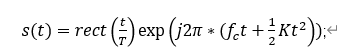可以很容易看出，线性调频信号的频率随着时间的推移而增大，而LFM信号的w满足式子：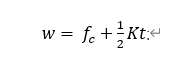可以看到，w和t满足线性关系，其中K为w的变化率，fc则是w的初值。
二、理论分析
1.高斯白噪声
由于我们加入的是高斯白噪声信号，高斯白噪声信号的功率谱密度在各个频段都是一个恒定的值，即满足N~(μ，σ2)，其中σ2表示噪声的平均功率；
2.设置匹配滤波器
匹配滤波器的公式：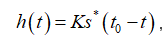下面我们来简单推到一下匹配滤波器：
我们先进行卷积运算：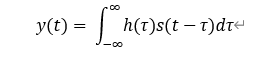信噪比：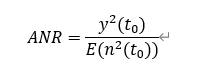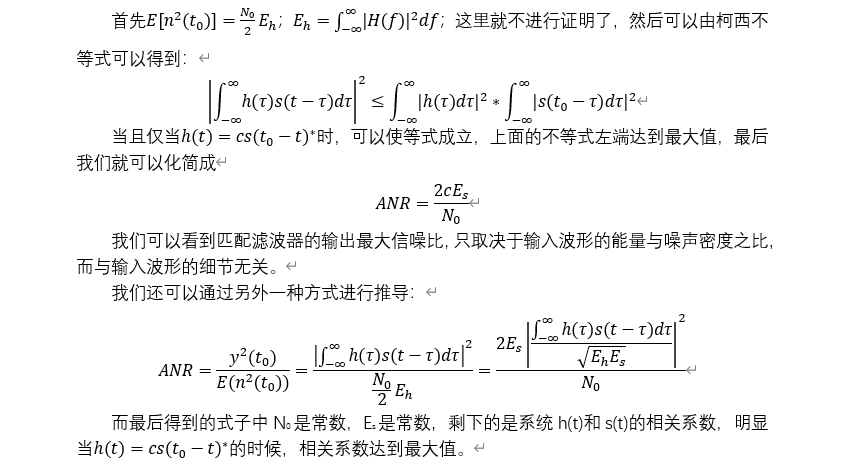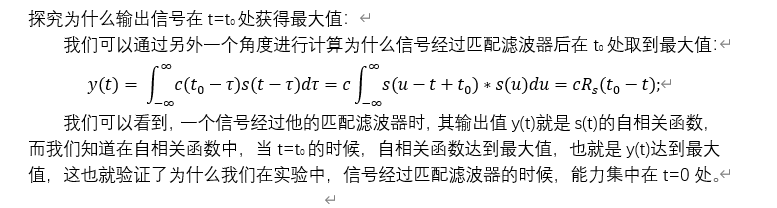最后的小结：
所以我们利用匹配滤波器的作用是什么，其实我们利用匹配滤波器不是为了还原这个波形，我们需要的，是得到这个信号的部分信息；很明显利用匹配滤波器，我们可以使LFM信号在接受时，形成脉冲，并且可以获取LFM的时间信息，这样我们就可以得到我们需要的测量值。
本文章为读者的课程设计笔记，如果有错误的地方，希望大家指出。


展开全文• 关于匹配滤波器的原理以及其matlab实现方式的详细过程 关于匹配滤波器的原理以及其matlab实现方式的详细过程
• 最近，我遇到了一个这样的...匹配滤波(match filter)是最佳线性滤波器的一种，该滤波器的准则是使输出信号的信噪比最大。常用于雷达、声呐等接收机的回波信号处理中，也可用于模式识别，相似度测试等。在下文中，...最近，我遇到了一个这样的问题。分析主动式声呐的回波信号，估算海底的深度，且声呐的发射信号波形已知。由于环境背景噪声的影响，往往不能直接从回波信号中读取出有关海底深度的到时信息。此时，就需要用到匹配滤波技术。匹配滤波(match filter)是最佳线性滤波器的一种，该滤波器的准则是使输出信号的信噪比最大。常用于雷达、声呐等接收机的回波信号处理中，也可用于模式识别，相似度测试等。在下文中，我们将介绍匹配滤波的基本理论，并给出基于matlab语言的主动式声呐测量海水深度的实例。匹配滤波的基本原理假设理想点目标与探测器(声呐或者雷达)的相对距离为，为了探测这个目标，探测器发射信号，发射波(声波或者电磁波)以速度向四周传播，经过时间后发射波到达目标，照射到目标上的发射波可写成：。发射波与目标相互作用，一部分发射波被目标散射，反射波为，其中为目标的散射截面，反映目标对发射波的散射能力。再经过时间后，接收机接收到的信号为。如果将探测器与目标看作一个系统，便得到如图1所示的线性时不变(Linear Time Invariant, 简称LTI)系统。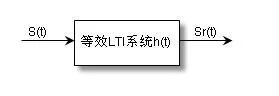图 1 等效LTI系统等效LTI系统的冲击响应可以写为：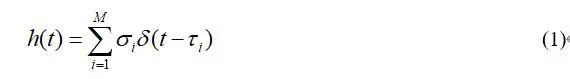其中，是目标的个数，是不同目标的散射截面，是发射波在目标与探测器间往返一次的时间，即：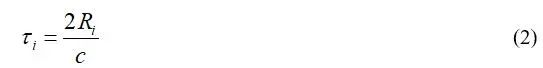式中，是第个目标与探测器之间的距离。发射信号经过该LTI系统，得到输出信号，即回波信号：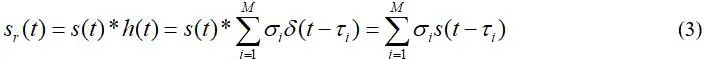那么，该如何从回波信号中提取出目标的特性信息(相对距离和散射特性)呢？常用的方法是让回波信号通过发射信号的匹配滤波器，如图2所示。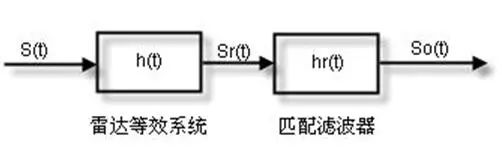图 2 回波信号处理的匹配滤波器为：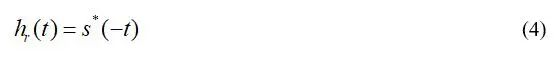由此可知，发射信号的匹配滤波器是其时间反序信号的共轭，由于发射信号都是实信号。所以发射信号对应的匹配滤波器是其时间反序信号。令回波信号通过匹配滤波器，可得：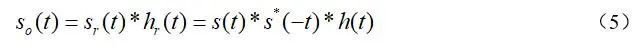对上式作傅立叶变换：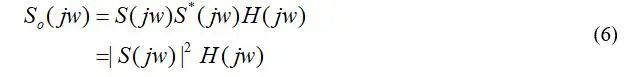如果选取合适的，使它的幅频特性为常数，那么式(6)可以写为：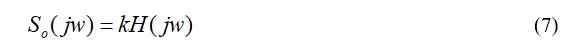其傅立叶反变换为：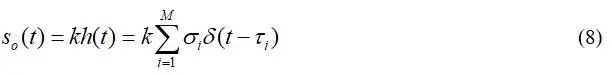中已经包含了目标的特性信息(距离和散射信息)，这就是匹配滤波的工作原理。匹配滤波的关键点在于要保证发射信号的幅频为常数。比较常用的发射信号是线性调频(Linear Frequency Modulation, 简称LFM)脉冲信号，关于发射信号的具体形式，可以参考博客SAR成像(一)，而且博客中还给出了一个理想的仿真实例。声呐测量海水深度问题描述用主动声呐进行海底深度测量，已知发射信号为LFM脉冲，中心频率为100 kHz，带宽为10 kHz，持续时间为1 ms。发射完后就开始接收海底反射回波，回波信号的采样率为500 kHz，数据为data3，试估计海底深度，海水声速度取1500 m/s。利用上文介绍的匹配滤波，我们就可以估算海底的深度。根据参数，可以得到发射信号的时域波形和频谱，如图3所示。接收机得到的回波波形如图4所示，信号中还有大量的随机噪声，导致在信号中没有占优的振幅出现，无法提取出和海底深度有关的到时信息。图5给出了回波信号通过匹配滤波器后的脉冲压缩波形，在0.06 s处有一个很明显的振幅尖峰出现，即是与海底深度有关的到时信息，最后估算的海底深度为46.3425 m。这个实例表明，匹配滤波在回波信号处理中是必不可少的一种技术。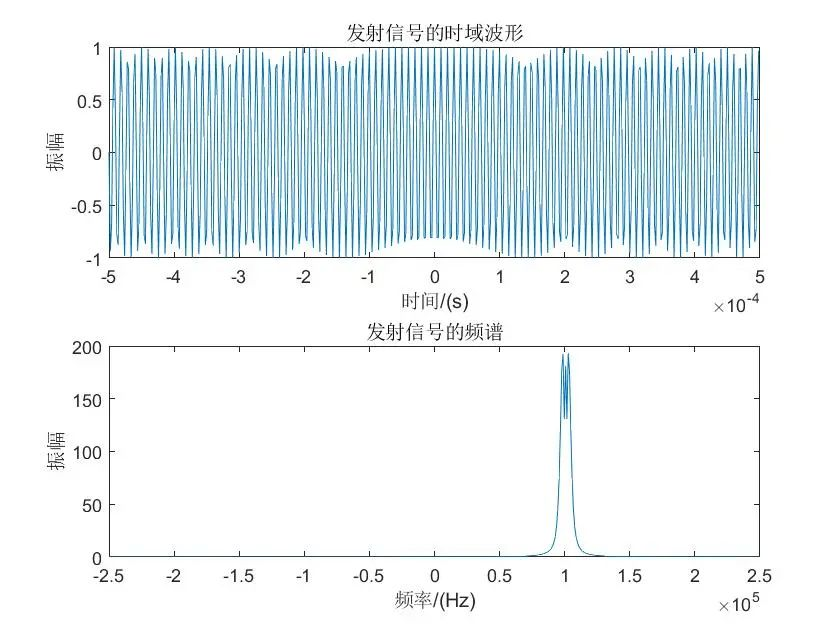图 3 发射信号的时域波形和频谱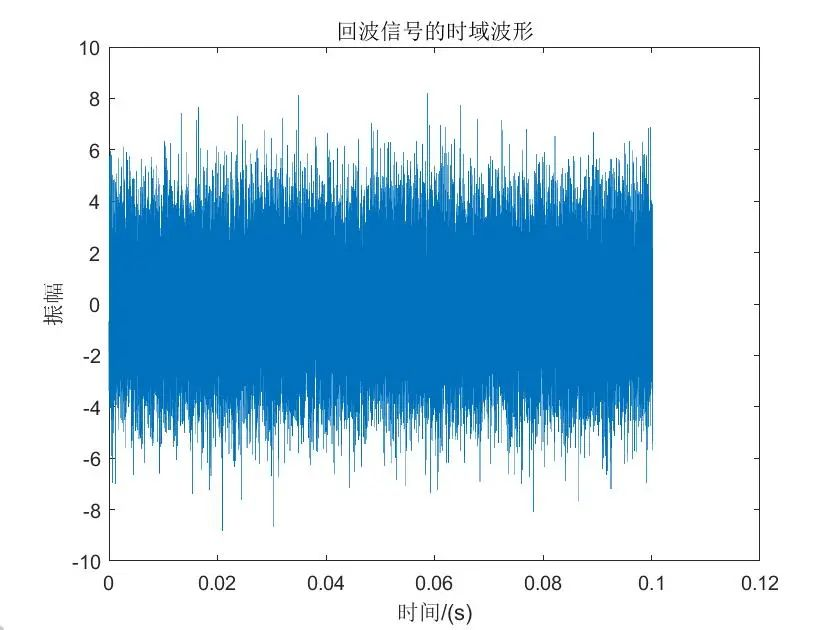图 4 回波信号的时域波形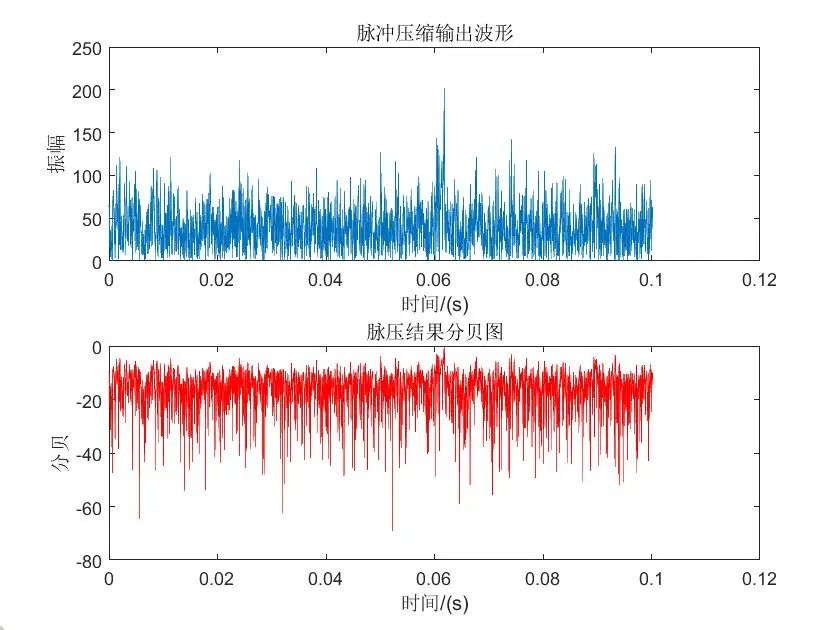图 5 脉冲压缩输出波形和分贝图matlab代码%% 匹配滤波实例：从主动式声呐的回波信号中提取海底深度load data3.mat;        % 加载回波文件Npt = length(data3);   % 回波信号的长度Fs = 500*1e3;          % 回波信号的采样率dt = 1/Fs;             % 回波信号的时间域采样间隔fm = 100*1e3;          % 发射信号的中心频率tao = 0.001;           % 发射信号脉冲持续时间B = 10*1e3;            % 频带宽度K = B/tao;             % 调频斜率t0 = -tao/2:dt:tao/2-dt; % 发射信号的时间向量v = 1500;              % 海水中的声波速度St0 = exp(1i*pi*(2*fm*t0+K.*(t0.^2)));  % 发射的脉冲信号% 绘制发射信号的时域波形和其频谱figure(1)subplot(211);plot(t0,real(St0));xlabel('时间/(s)');ylabel('振幅');title('发射信号的时域波形')X=fftshift(fft(St0));f=linspace(0,Fs,length(t0))-Fs/2;subplot(212);plot(f,abs(X));xlabel('频率/(Hz)');ylabel('振幅');title('发射信号的频谱')% 绘制回波信号的时域波形figure(2)plot(0:dt:(Npt-1)*dt, data3);xlabel('时间/(s)');ylabel('振幅');title('回波信号的时域波形');% 匹配滤波h=exp(-1i*pi*(2*fm*t0+K.*t0.^2)); % 匹配滤波器output = ifft((fft(h,Npt).*fft(data3,Npt))); % 作匹配滤波% 绘制脉冲压缩输出波形和脉压结果分贝图figure(3)subplot(211);plot(0:dt:Npt*dt-dt,abs(output));  % 取绝对值或者取实部都可以，不影响最后计算结果xlabel('时间/(s)');ylabel('振幅');title('脉冲压缩输出波形');subplot(212);plot(0:dt:Npt*dt-dt,db(abs(output)/max(abs(output))),'r');xlabel('时间/(s)');ylabel('分贝');title('脉压结果分贝图');[~,I] = max(output);depth = v*I*dt/2;str = sprintf('海水深度为%fm.', depth);disp(str);PS：完整的代码例子和数据已经整理到我的百度企业云盘中，需要的同学可以从以下分享链接(有效期7天)下载：https://eyun.baidu.com/s/3dGoLDzN参考资料SAR成像(一): https://blog.csdn.net/a1367666195/article/details/105979333

展开全文• 最近，我遇到了一个这样的...匹配滤波(match filter)是最佳线性滤波器的一种，该滤波器的准则是使输出信号的信噪比最大。常用于雷达、声呐等接收机的回波信号处理中，也可用于模式识别，相似度测试等。在下文中，...
• 在信号与系统的信号矢量空间一章提到了匹配滤波器的应用，尝试用matlab将典型例题做仿真。 例题 例6-7在测距系统中，发送信号s(t),以匹配滤波器接收回波信号,利用滤波器输出信号峰值出现的时间折算目标距离。如果有...
• 背景   雷达接收机在接收回波信号的同时，不可避免地会遇到噪声，同时还会受到各种干扰，如各种分布物体产生的杂波干扰、敌方施放的噪声调制干扰等。为了选出有用目标，同时抑制各种噪声和干扰，...匹配滤波器是在信号处理 卷积
• 匹配滤波器的仿真实验报告(大学电子信息工程专业)实验一 匹配滤波器的仿真验证一、实验目的：利用matlab验证匹配滤波器的特性二、实验要求：设二进制数字基带信号s(t)=∑aang(t-nTs)，加性高斯白噪声的功率谱密度为0...
• 摘 要 在现代通信系统中由于信号中经常混有各种复杂成分所以很多信号分 析都是基于滤波器而进行的而数字滤波器是通过数值运算实现滤波具有处理 精度高稳定灵活不存在阻抗匹配问题可以实现模拟滤波器无法实现的特 殊...
• 关于匹配滤波器的原理以及其matlab实现方式的详细过程 匹配滤波器的简单实现
• 本讲使用两个DDS产生待滤波的信号和 matlab 产生带滤波信号，结合FIR滤波器搭建一个信号产生及滤波的系统，并编写 testbench 进行仿真分析，下一讲 开始编写 verilog 代码设计FIR滤波器，不再调用IP核。本讲很多地方...
• 一个扩频发射接收机的matlab仿真程序用匹配滤波器的方法同步 一个扩频发射接收机的matlab仿真程序用匹配滤波器的方法同步
• 匹配滤波器的实验，带MATLAB程序，雷达LFM应用
• 本资源提供了空间信号处理中基本的空间匹配滤波器的实现以及当存在干扰较大时，往往会影响我们提取有用的信号，通过锥化截取滤波属于一种简要的非自适应的方式来客服干扰，从而获取我们的期望信号。
• ***************************************** 利用matlab程序设计的匹配滤波器的功能源码* *****************************************...

# 匹配滤波器matlabmatlab 订阅